Topics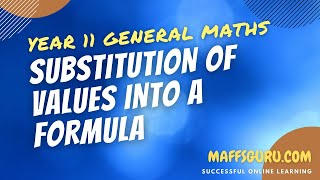###### Linear relations and equations
Free to view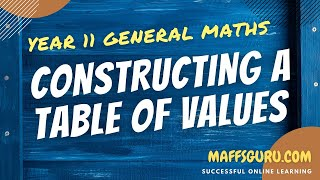###### Linear relations and equations
Free to view###### Linear relations and equations
Free to view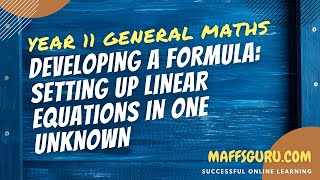###### Linear relations and equations
Free to view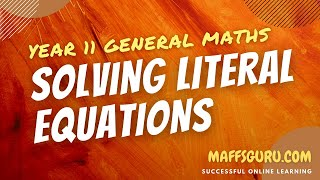###### Linear relations and equations
Free to view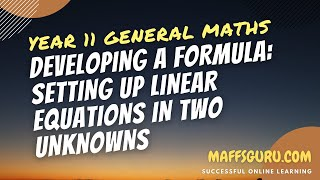###### Linear relations and equations
Free to view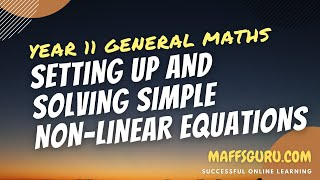###### Linear relations and equations
Free to view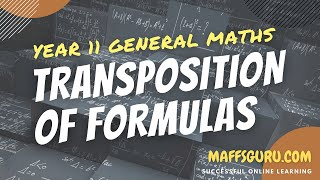###### Linear relations and equations
Subscribers Only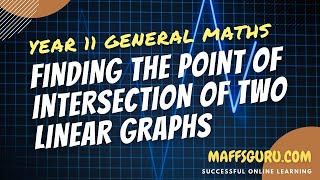###### Linear relations and equations
Subscribers Only###### Linear relations and equations
Subscribers Only###### Linear relations and equations
Subscribers Only###### Linear relations and equations
Subscribers Only
Select a topic
These are the videos which are available in this chapter. You will notice that some are free and some are for subscribers only. Making and hosting these videos costs a little bit of money. To help pay for the hosting fees I have no choice but to hide some of the videos and make them for subscribers only.